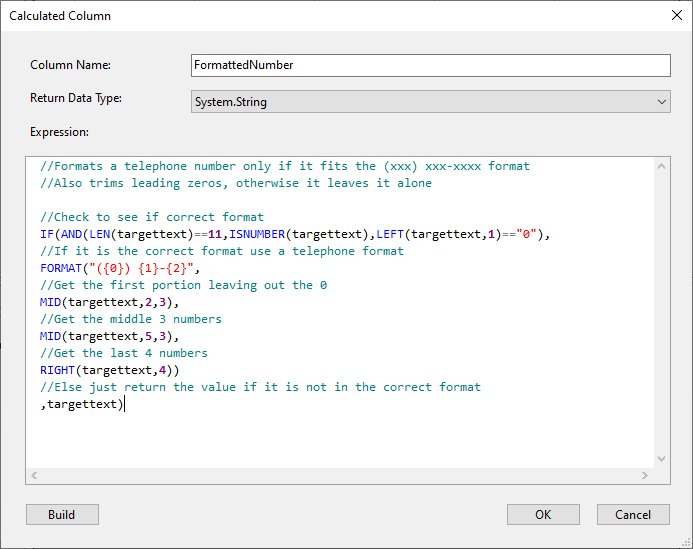User Guide

# Calculated Columns

Calculated Columns are new columns added to the row that are a calculation based on data from the current row. These allow you to easily transform values via the in-built functions to create new row values.

These Calculated columns can then be used in the Schema Map like any other column and mapped to your target.

To add a new calculated column press the Delete Calculated Column (Fx) button in the mini-toolbar or press the shortcut keys ALT+C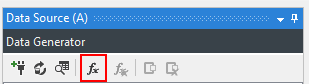This opens the Calculated Column configuration dialog where you give your Calculated Column a Name, return Data Type and expression.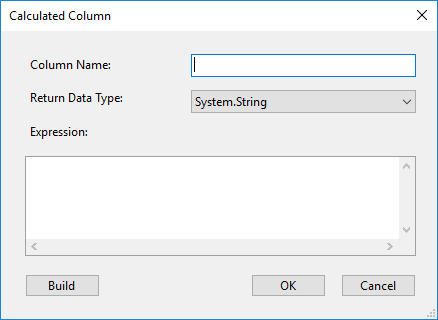The Name must be unique within your schema, you cannot use an existing name from your Data Source Schema. The Expression is the data result of the column for the given row, this expression is a C# value however we have made this more Excel like with the introduction of many functions for typical scenarios.

For example below we use the FORMAT function to create an email address, replacing the row ID value for each row. (testuser1@simego.com, testuser2@simego.com, testuser3@simego.com etc.)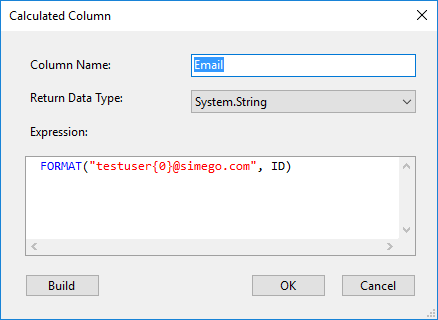This is the same as writing the following code in Dynamic Columns. With Calculated Columns this is much quicker and easier.

``````public string Email
{
get
{
return string.Format("testuser{0}@simego.com", ID);
}
}
``````

Pressing the Build button will compile your Calculated Column and verify that the syntax is correct.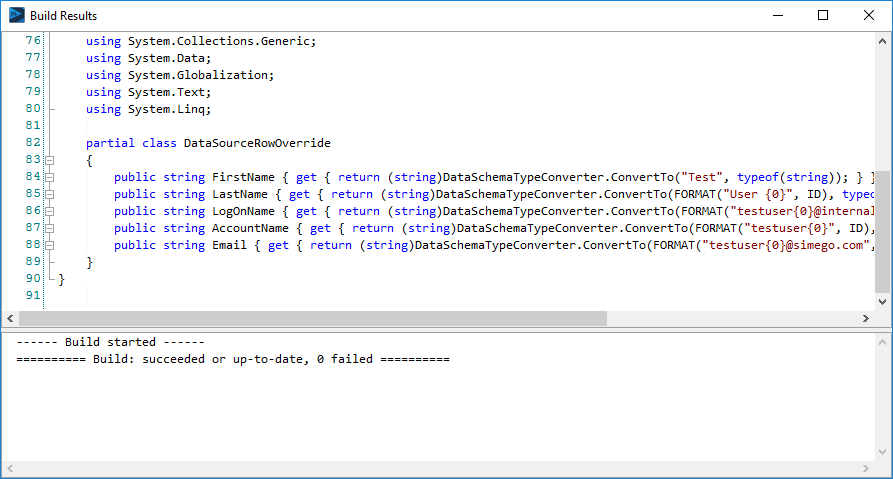## Delete Calculated Column

To delete a Calculated Column, first select the column in the Data Source and then press the Delete Calculated Column button in the mini-toolbar.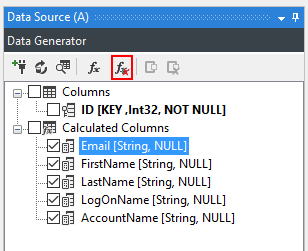## Functions

Array

A list of all functions relating to arrays.

Calculations

A list of all functions relating to calculations.

DateTime

A list of all functions relating to DateTime.

File

A list of all functions relating to files.

Logic

A list of all functions relating to logic.

Lookup

A list of all functions relating to lookups.

Miscellaneous

A list of all other functions. -->

Numbers

A list of all functions relating to numbers.

Strings

A list of all functions relating to strings.

To show the extent it is possible to manipulate and manage a complex transformation we can use the following as an example requirement:

A telephone number formatting function will not only handle the leading zeros, but if there are exactly 10 numeric characters in the source, it will also format it as (xxx) xxx-xxxx. If there are not exactly 10 character in the source the function will simply populate the raw value from your source.

``````
IF(AND(LEN(targettext)==11,ISNUMBER(targettext),LEFT(targettext,1)=="0"),
FORMAT("({0}) {1}-{2}",
MID(targettext,2,3),
MID(targettext,5,3),
RIGHT(targettext,4))
,targettext)
``````